# 如何排序？

NBA总决赛正在如火如荼的进行，老詹也正朝着他的第5个总亚军前进着。假设骑士队队员在运动场上排列成一队，如图所示，所有队员已经站好，准备热身，现在需要按身高从低到高 为队员们排队（最矮的站在左边），给他们照一张集体照，应该怎么排队呢？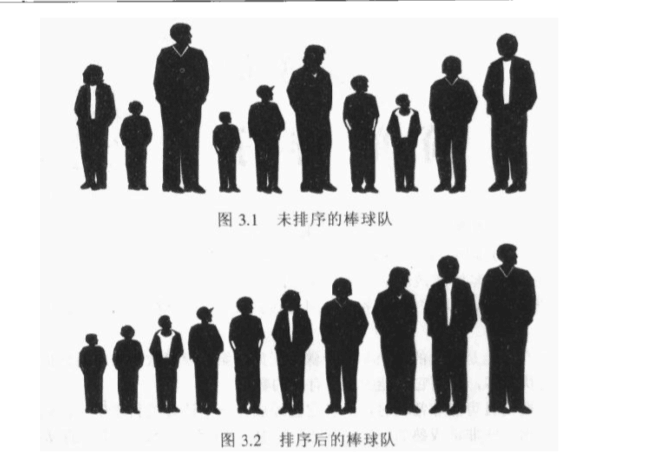1. 比较两个数据项

2. 交换两个数据项，或复制其中一项

# BUBBLE 冒泡排序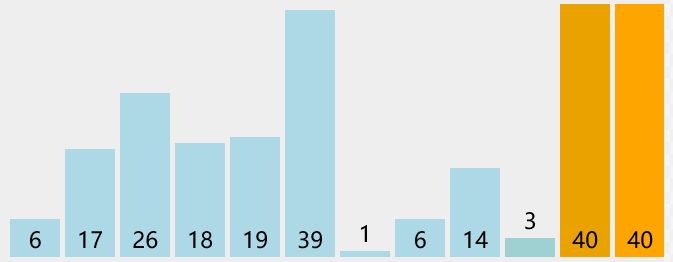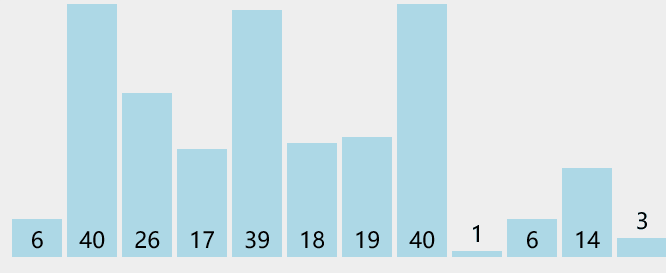1. 比较两个数字

2. 如果左边的数字大，则交换两个数字的位置

3. 向右移动一个位置，比较下两个数字

沿着这个顺序比较下去，一直比较到最右端，虽然没有把所有的数字排好序，但是，数字中最大的那一个已经排放在最右边了，这个是一轮比较下来 可以确定的结果。

下一轮，我们继续从最左边开始。

这也是这个算法被称为冒泡排序的原因：因为在算法执行的时候，最大的数据项 总是 “冒泡”到数组的顶端【数组的排列规则，从左到右0-N】。

```代码实现：
\$arr=array(1,43,54,62,21,66,32,78,36,76,39);
function bubbleSort(\$arr)
{
\$len=count(\$arr);
//该层循环控制 需要冒泡的轮数
for(\$i=1;\$i<\$len;\$i++)
{ //该层循环用来控制每轮 冒出一个数 需要比较的次数
for(\$k=0;\$k<\$len-\$i;\$k++)
{
if(\$arr[\$k]>\$arr[\$k+1])
{
\$tmp=\$arr[\$k+1];
\$arr[\$k+1]=\$arr[\$k];
\$arr[\$k]=\$tmp;
}
}
}
return \$arr;
}```

# SELECT 选择排序

## 理解一下选择排序的原理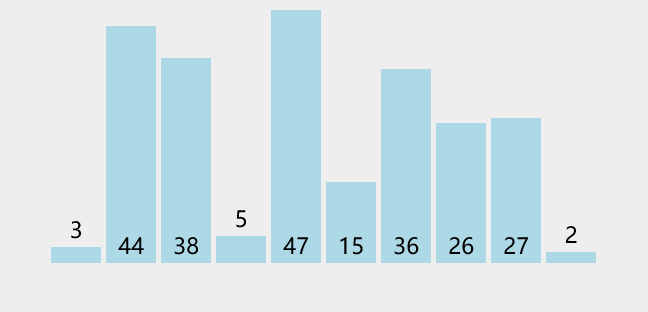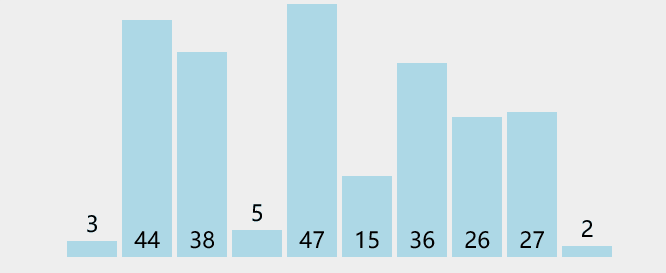## 选择排序的代码实现

```//选择排序法
//实现思路 双重循环完成，外层控制轮数，当前的最小值。内层 控制的比较次数
function select_sort(\$arr) {
//\$i 当前最小值的位置， 需要参与比较的元素
for(\$i=0, \$len=count(\$arr); \$i<\$len-1; \$i++) {
//先假设最小的值的位置
\$p = \$i;
//\$j 当前都需要和哪些元素比较，\$i 后边的。
for(\$j=\$i+1; \$j<\$len; \$j++) {
//\$arr[\$p] 是 当前已知的最小值
if(\$arr[\$p] > \$arr[\$j]) {
//比较，发现更小的,记录下最小值的位置；并且在下次比较时，应该采用已知的最小值进行比较。
\$p = \$j;
}
}
//已经确定了当前的最小值的位置，保存到\$p中。
//如果发现 最小值的位置与当前假设的位置\$i不同，则位置互换即可
\$tmp = \$arr[\$p];
\$arr[\$p] = \$arr[\$i];
\$arr[\$i] = \$tmp;
}
//返回最终结果
return \$arr;
}```

# INSERT 插入排序

## 插入排序原理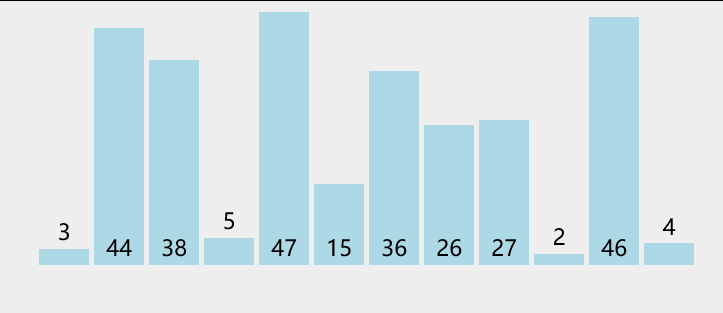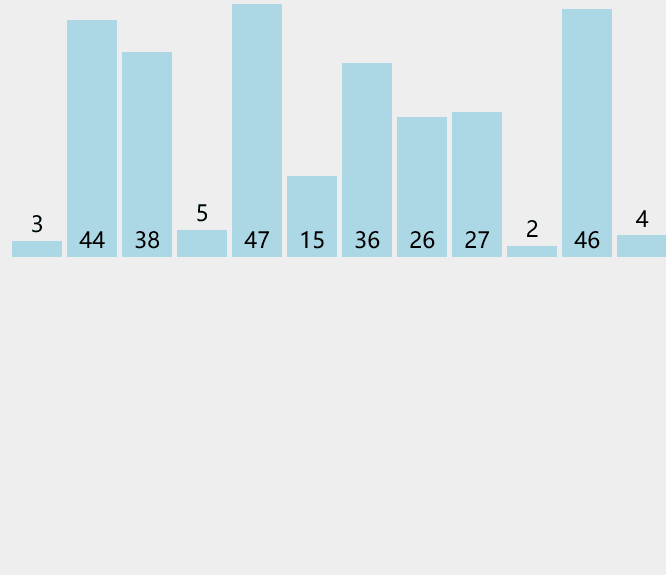## 用插入排序提醒老詹吧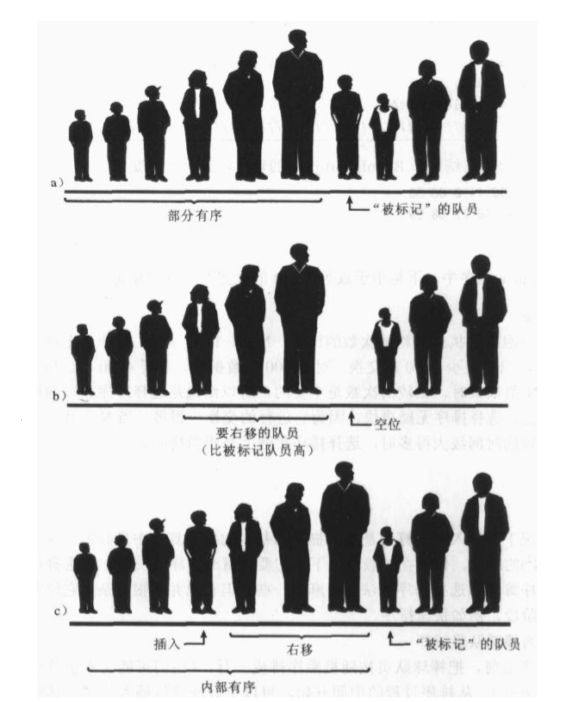## 插入排序的核心代码：

```function insertSort(\$arr) {
\$len=count(\$arr);
for(\$i=1, \$i<\$len; \$i++) {
\$tmp = \$arr[\$i];
//内层循环控制，比较并插入
for(\$j=\$i-1;\$j>=0;\$j--) {
if(\$tmp < \$arr[\$j]) {
//发现插入的元素要小，交换位置，将后边的元素与前面的元素互换
\$arr[\$j+1] = \$arr[\$j];
\$arr[\$j] = \$tmp;
} else {
//如果碰到不需要移动的元素，由于是已经排序好是数组，则前面的就不需要再次比较了。
break;
}
}
}
return \$arr;
}```

## 插入排序的效率（有趣）

1+2+3+…+N-1=N*（N-1）/2 次比较；

# MERGE 归并排序

## 感受归并排序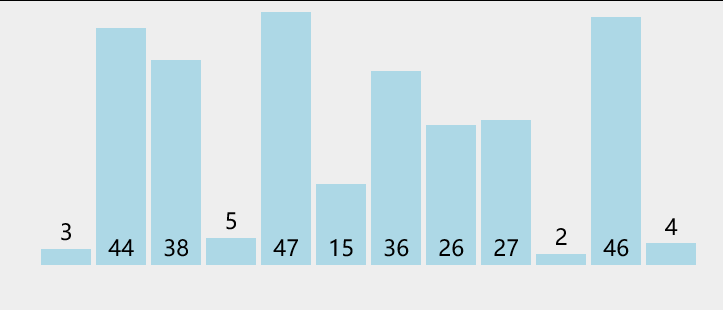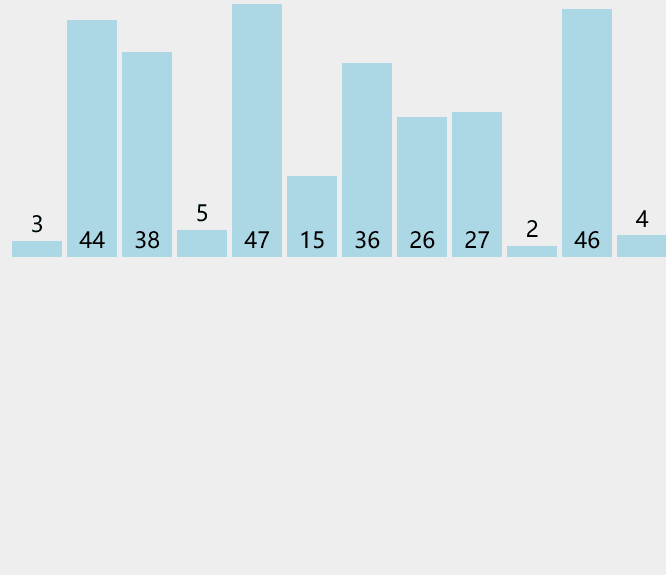```<?php
\$arrStoreList = array(3,2,4,1,5);
\$sort = new Merge_sort();
\$sort->stableSort(\$arrStoreList, function (\$a, \$b) {    // function (\$a, \$b)匿名函数
return \$a < \$b;
});
//静态调用方式也行
/*Merge_sort:: stableSort(\$arrStoreList, function (\$a, \$b) {
return \$a < \$b;
});*/
print_r(\$arrStoreList);

class Merge_sort{

public static function stableSort(&\$array, \$cmp_function = 'strcmp') {
//使用合并排序
self::mergeSort(\$array, \$cmp_function);
return;
}
public static function mergeSort(&\$array, \$cmp_function = 'strcmp') {
// Arrays of size < 2 require no action.
if (count(\$array) < 2) {
return;
}
// Split the array in half
\$halfway = count(\$array) / 2;
\$array1 = array_slice(\$array, 0, \$halfway);
\$array2 = array_slice(\$array, \$halfway);
// Recurse to sort the two halves
self::mergeSort(\$array1, \$cmp_function);
self::mergeSort(\$array2, \$cmp_function);
// If all of \$array1 is <= all of \$array2, just append them.
//array1 与 array2 各自有序;要整体有序，需要比较array1的最后一个元素和array2的第一个元素大小
if (call_user_func(\$cmp_function, end(\$array1), \$array2) < 1) {
\$array = array_merge(\$array1, \$array2);

return;
}
// 将两个有序数组合并为一个有序数组：Merge the two sorted arrays into a single sorted array
\$array = array();
\$ptr1 = \$ptr2 = 0;
while (\$ptr1 < count(\$array1) && \$ptr2 < count(\$array2)) {
if (call_user_func(\$cmp_function, \$array1[\$ptr1], \$array2[\$ptr2]) < 1) {
\$array[] = \$array1[\$ptr1++];
} else {
\$array[] = \$array2[\$ptr2++];
}
}
// Merge the remainder
while (\$ptr1 < count(\$array1)) {
\$array[] = \$array1[\$ptr1++];
}
while (\$ptr2 < count(\$array2)) {
\$array[] = \$array2[\$ptr2++];
}
return;
}
}
?>```7条评论